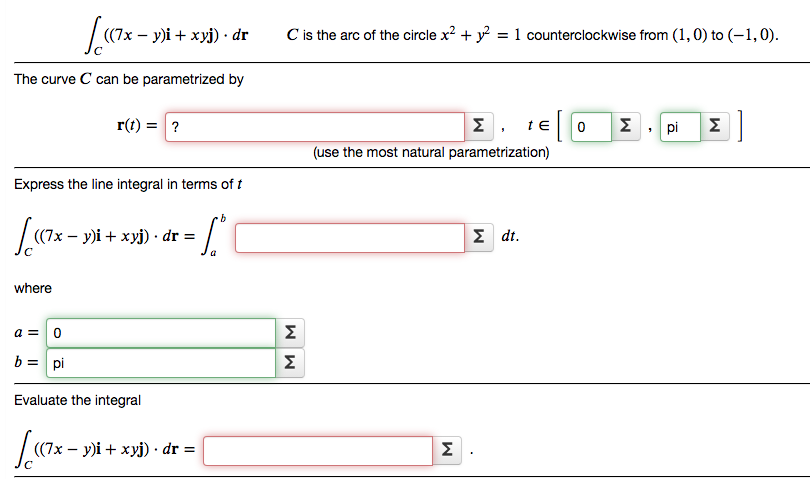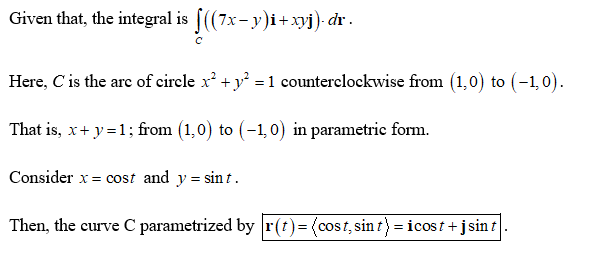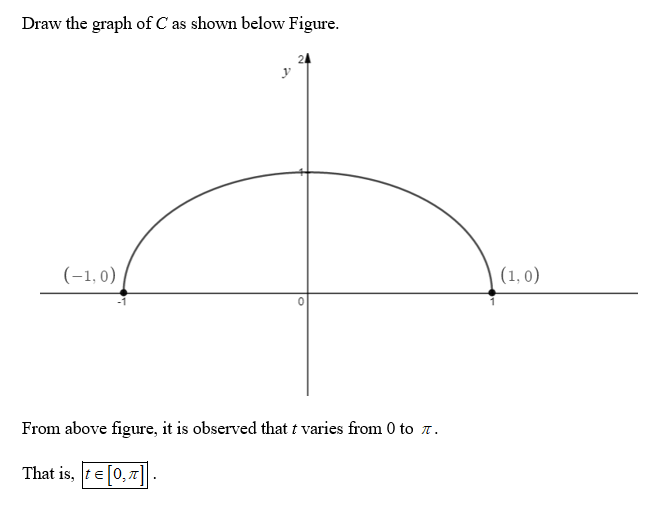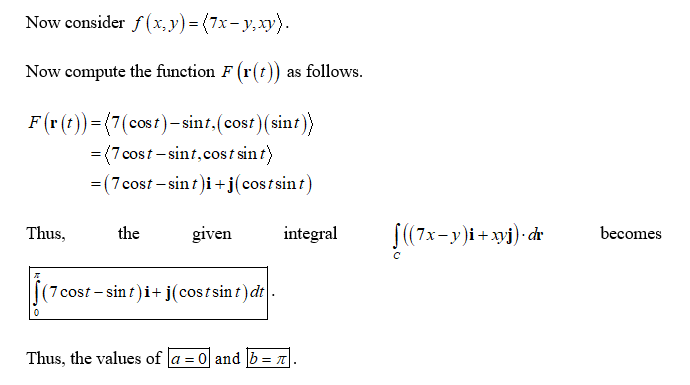# (7х — у)і + хуј) . drC is the arc of the circle x? + y = 1 counterclockwise from (1,0) to (-1,0).%3DThe curve C can be parametrized byr(t) = ?ΣteΣ.piΣ|(use the most natural parametrization)Express the line integral in terms of t((7x – y)i + xyj) · dr =Σ dt .whereΣb = piΣEvaluate the integral(7х — у)і + хуј) dr %3DΣ

Question
4 views

Evaluate the line integralhelp_outlineImage Transcriptionclose(7х — у)і + хуј) . dr C is the arc of the circle x? + y = 1 counterclockwise from (1,0) to (-1,0). %3D The curve C can be parametrized by r(t) = ? Σ te Σ.pi Σ| (use the most natural parametrization) Express the line integral in terms of t ((7x – y)i + xyj) · dr = Σ dt . where Σ b = pi Σ Evaluate the integral (7х — у)і + хуј) dr %3D Σ fullscreen
check_circle

Step 1Step 2Step 3...

### Want to see the full answer?

See Solution

#### Want to see this answer and more?

Solutions are written by subject experts who are available 24/7. Questions are typically answered within 1 hour.*

See Solution
*Response times may vary by subject and question.
Tagged in